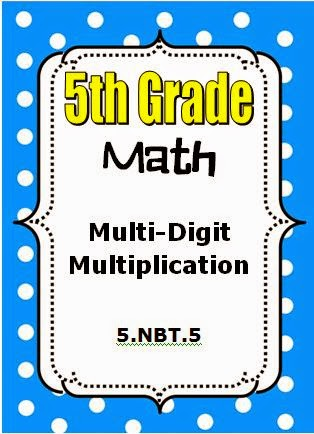## Sunday, September 28, 2014

### by Hilda Ratliff

Grade 5                                                       Click here to preview.This resource gives a step by step procedure for multiplying multi-digit numbers using the standard algorithm. The carries are recorded in each partial product so that the student can actually see the complete product of each multiplication being performed.

This resource may be used to introduce the standard algorithm of multiplication. Students will find products of two 3-digit numbers, a 4-digit number and a 2-digit number, and a 4-digit number and a 3-digit number. A template is used in the first activity for the student to become accustomed to recording and adding partial products.

The resource may be used for remediation after multiplication of multi-digit numbers has been taught.

Included in this Resource:

1. Handout with guided practice, specific procedural instructions, and independent practice – 4 pages
2. Handout with guided practice and independent practice – 2 pages
3. Group activity with 6 task cards